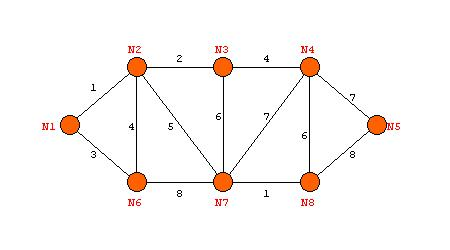Prim's Algorithm: An Interactive ExampleNOTE: The example graph below is used to show how Kruskal's Algorithm works for the determining of the minimum spanning tree (MST).   It is highly recommended, in order for you to see the difference between Kruskal's Algorithm and Prim's Algorithm, that you draw the below graph for the Prim applet, and go through it step by step.Kruskal's Algorithm
 Using the above graph, here are the steps to the MST, using Kruskal's Algorithm: N1 to N2 - cost is 1 - add to tree N7 to N8 - cost is 1 - add to tree N2 to N3 - cost is 2 - add to tree N1 to N6 - cost is 3 - add to tree N2 to N6 - cost is 4 - reject because it forms a circuit N3 to N4 - cost is 4 - add to tree N2 to N7 - cost is 5 - add to tree N3 to N7 - cost is 6 - reject because it forms a circuit N4 to N8 - cost is 6 - reject because it forms a circuit N4 to N7 - cost is 7 - reject because it forms a circuit N4 to N5 - cost is 7 - add to tree We stop here, because n -1 edges have been added.  We are left with the minimum spanning tree, with a total weight of 23.

Click here to see a graphical solution of Kruskal's Algorithm.
Or move on to the next page to go directly to the next section.